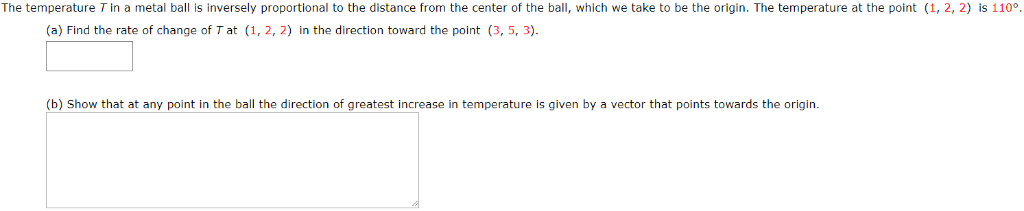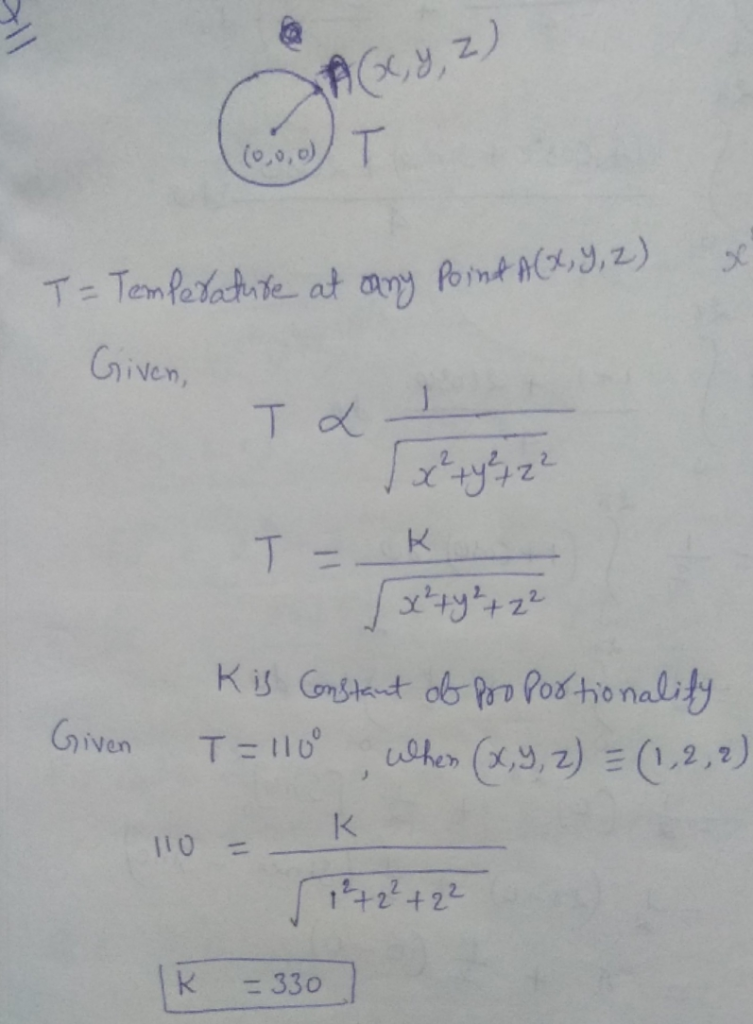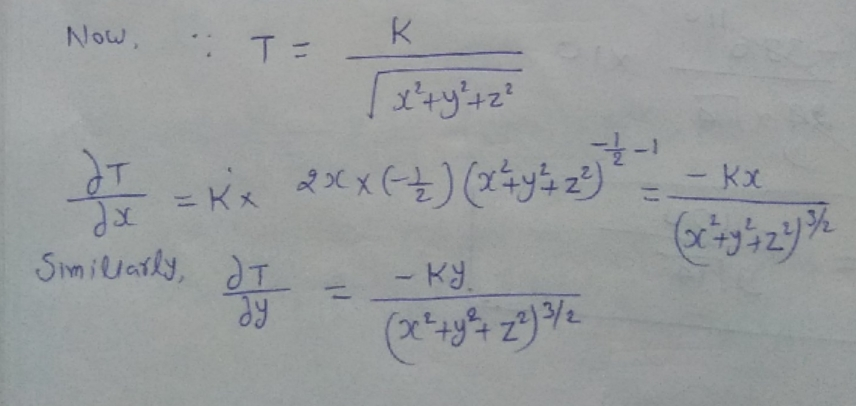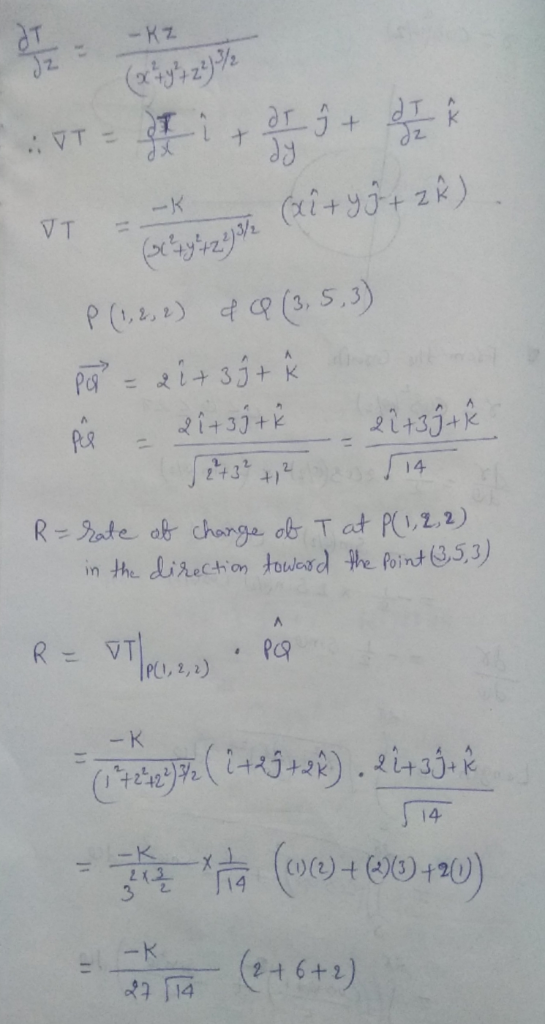Homework Help Question & Answers

The temperature T in a metal ball is inversely proportional to the distance from the center of the ball, which we take to be the origin. The temperature at the point (1, 2, 2) is 110° (a) Find the ra...The temperature T in a metal ball is inversely proportional to the distance from the center of the ball, which we take to be the origin. The temperature at the point (1, 2, 2) is 110° (a) Find the rate of change of T at (1, 2, 2) in the direction toward the point (3, 5, 3) (b) Show that at any point in the ball the direction of greatest increase in temperature is given by a vector that points towards the originAdd Answer of: The temperature T in a metal ball is inversely proportional to the distance from the center of the ball, which we take to be the origin. The temperature at the point (1, 2, 2) is 110° (a) Find the ra...
More Homework Help Questions Additional questions in this topic.

• 6. (extra credit) Find the center of mass of a region inside a circle of radius a if the density at any point is proportional to its distance from the center. (Either compute the center, or guess it...

Need Online Homework Help?

Get FREE EXPERT Answers
WITHIN MINUTES
Related Questions